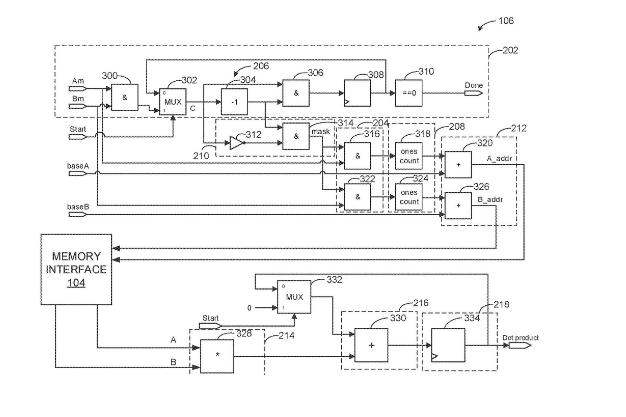# DOT PRODUCT CALCULATORS AND METHODS OF OPERATING THE SAMEMethods, apparatus, systems and articles of manufacture to perform dot product calculations using sparse vectors are disclosed. An example dot product calculator includes a first logic AND gate to perform a first logic AND operation with a first input vector and a second input vector, the first logic AND gate to output a control vector; a second logic AND gate to perform a second logic AND operation with a difference vector and an inverse of the control vector, the second logic AND gate to output a mask vector; a third logic AND gate to output a first vector; a first counter to generate a first ones count based on a first total number of ones of the first vector; a fourth logic AND gate to output a second vector; a second counter to generate a second ones count; and a multiplier to generate a product.